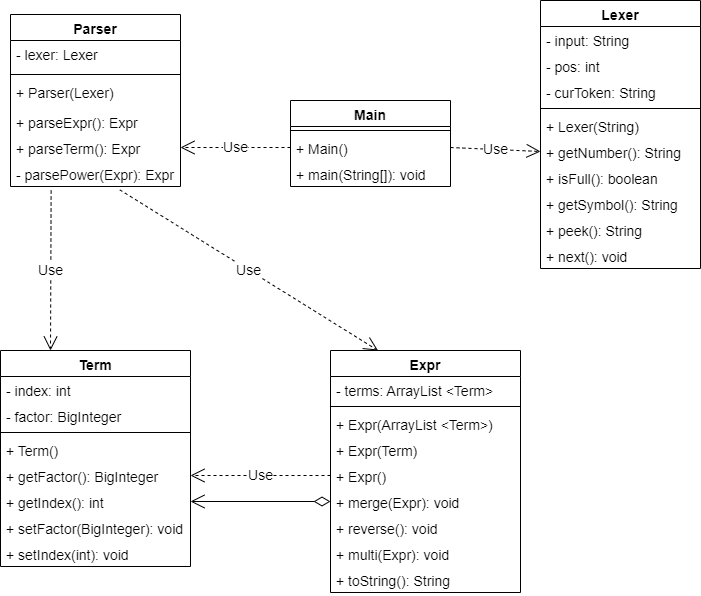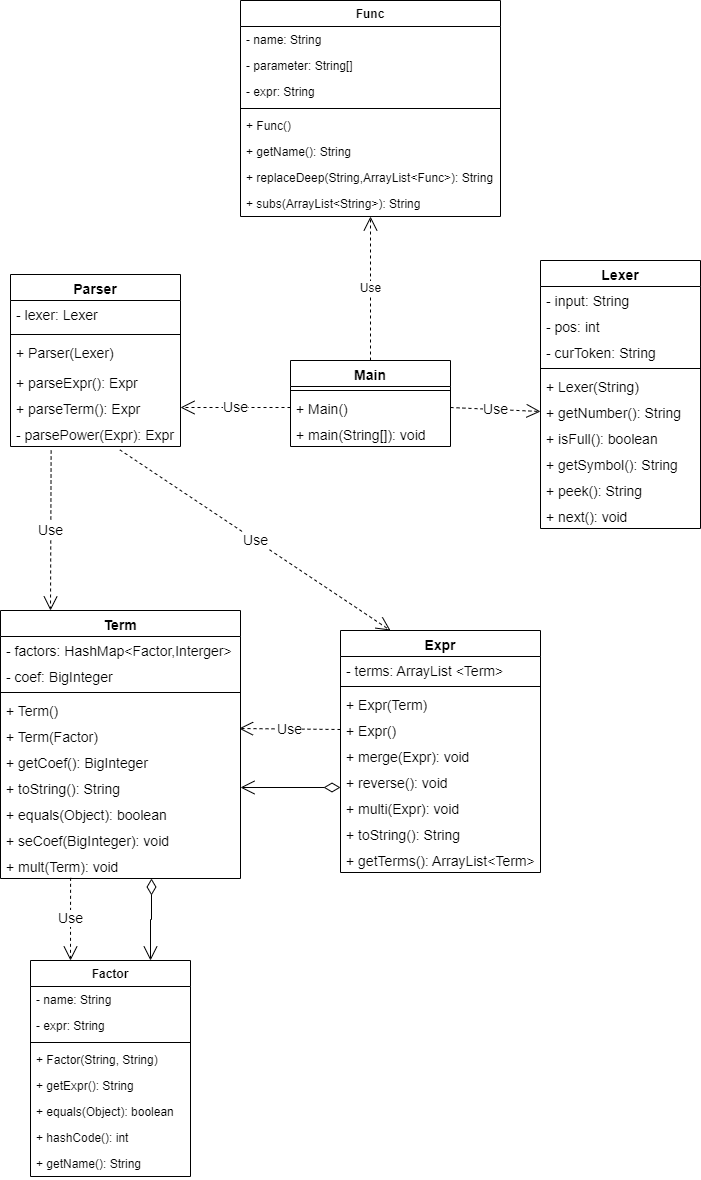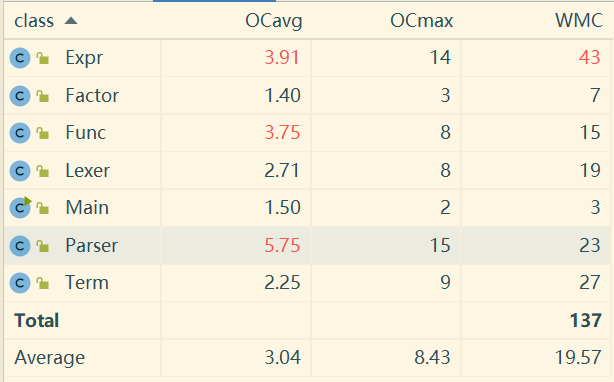# OO第一单元总结

## 代码架构设计

### 第一次作业### 第二次作业• 把自定义函数的代换作为读入的预处理，通过一个独立的类用replace方法暴力解决
• 把自定义函数作为解析的一部分，重构Parser的功能，实现自定义函数的代换

• 完全符合开闭原则，预处理的过程与之后的化简过程完全分开，方便调试
• 实现很简洁，整个Func类只有80行
• 具有一定的可扩展性，只要函数名和变量名已知，就可以满足需求

### 第三次作业

$sin^2(x)+cos^2(x)=1$

## 圈复杂度分析### Expr

Expr类中的方法圈复杂度大多比较正常，只有simplify方法一枝独秀，simplify方法是用来完成三角优化的方法，对于每次合并完的Expr都会调用simplify进行三角优化。simplify方法之所以圈复杂度如此之高，是因为枚举Term和Term中因子的时候使用了多重循环，加之判断三角优化的条件十分复杂，导致循环中的分支判断也有很多，所以圈复杂度相当高。在实现这个方法之后，我也思考过对其进行优化和扩展。方法是将三角优化的判断单独作为一个私有方法来处理，这样不仅能够有效降低代码圈复杂度，还能具备良好的扩展性，进而实现更多的三角优化。不过受限于时间，我尚未实现。

void simplify() {
while (true) {
boolean isMerged = false;
for (int i = 0;i < terms.size(); i++) {
Term term1 = terms.get(i);
for (int j = 0;j < terms.size(); j++) {
Term term2 = terms.get(j);
BigInteger conFactor = getFactor(term1.getCoef(),term2.getCoef());
if (conFactor == null) {
continue;
}
HashMap<Factor,Integer> s = new HashMap<>();
HashMap<Factor,Integer> t1 = new HashMap<>();
HashMap<Factor,Integer> t2 = new HashMap<>();
HashMap<Factor,Integer> factors1 = term1.getFactors();
HashMap<Factor,Integer> factors2 = term2.getFactors();
for (Factor factor : factors1.keySet()) {
if (!factors2.containsKey(factor)) {
t1.put(factor,factors1.get(factor));
} else if (factors1.get(factor) <= factors2.get(factor)) {
s.put(factor,factors1.get(factor));
t2.put(factor,factors2.get(factor) - factors1.get(factor));
} else {
s.put(factor,factors2.get(factor));
t2.put(factor,factors1.get(factor) - factors2.get(factor));
}
}
for (Factor factor : factors2.keySet()) {
if (!factors1.containsKey(factor)) {
t2.put(factor,factors2.get(factor));
}
}
if (t1.size() == 1 && t2.size() == 1) {
Factor factor1 = t1.keySet().iterator().next();
Factor factor2 = t2.keySet().iterator().next();
if (factor1.getExpr().equals(factor2.getExpr())) {
if (t1.get(factor1) == 2 && t2.get(factor2) == 2) {
isMerged = true;
}
}
}
}
}
if (!isMerged) {
break;
}
}
}


### Func

Func类主要的圈复杂度问题在于replaceDeep方法。由于replaceDeep方法是直接对字符串进行解析，所以分支判断很多，造成了圈复杂度较高。由于replaceDeep方法需要递归调用，所以难以像simplify那样拆分函数，所幸replaceDeep方法并不冗长，只有30行，还是具备较好的可读性的。### Parser

Parser类主要的圈复杂度问题在于parseTerm方法。与Func类中的replaceDeep方法类似，parseTerm也是直接处理字符串的方法，因此parseTerm中也存在大量的分支判断。事实上，我认为parseTerm方法是整个代码最混乱、最危险的部分，我一直想要用更加清晰的方式来替代Parser+Lexer的组合，但是很难做到。由于表达式的复杂性，我们没法单单用 +-* 来分割表达式并且递归处理，还是需要依靠一点点的顺序读入、解析。当然，即使没法做大的改变，一些小的圈复杂度的优化还是可以做的，比如把一个分支中的代码全部放在一个私有方法中调用。## bug分析

### 第一次作业

Expr expr2 = expr;
for (int i = 1; i < index; i++) {
expr = expr * expr2;
}


### 第二次作业

f(y,x) = x + y
f(x,2)
f(x,x) = x + x //代入y = x
f(x,2) = 4 //代入x = 2


## 心得体会

posted @ 2022-03-25 17:38  hua-0x522  阅读(46)  评论(1编辑  收藏  举报